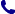• Kontakt
• Newsletter Zapisz się po informacje o nowościach i specjalnych ofertach. Administrator danych: ABE-IPS sp. z o.o.
Wybierz interesujące Cię kategorie
Wyrażam zgodę na przetwarzanie moich danych osobowych w celach marketingowych, tym samym akceptuję Regulamin Newslettera i Politykę prywatności.
Wyrażam zgodę na przesyłanie informacji handlowych drogą elektroniczną.
Wypisz się
• Klienci instytucjonalni
• Dostawa
• O nas
Dostęp on-line

# Książki

0.00 PLN
Schowek (0)
 Schowek jest pusty

## Probabilistic Methods in Geometry, Topology and Spectral Theory

 Autorzy Wydawnictwo American Mathematical Society Data wydania 01/12/2019 Wydanie Pierwsze Liczba stron 208 Forma publikacji książka w miękkiej oprawie Poziom zaawansowania Dla profesjonalistów, specjalistów i badaczy naukowych Język angielski ISBN 9781470441456 Kategorie Rachunek różniczkowy i formuły, Geometria, Topologia, Prawdopodobieństwo i statystyka
598.00 PLN (z VAT)
$134.52 / €128.21 / £111.30 / Produkt na zamówienie Dostawa 3-4 tygodnie Ilość Do schowka ## Opis książki This volume contains the proceedings of the CRM Workshops on Probabilistic Methods in Spectral Geometry and PDE, held from August 22-26, 2016 and Probabilistic Methods in Topology, held from November 14-18, 2016 at the Centre de Recherches Mathematiques, Universite de Montreal, Montreal, Quebec, Canada. Probabilistic methods have played an increasingly important role in many areas of mathematics, from the study of random groups and random simplicial complexes in topology, to the theory of random Schrodinger operators in mathematical physics. The workshop on Probabilistic Methods in Spectral Geometry and PDE brought together some of the leading researchers in quantum chaos, semi-classical theory, ergodic theory and dynamical systems, partial differential equations, probability, random matrix theory, mathematical physics, conformal field theory, and random graph theory. Its emphasis was on the use of ideas and methods from probability in different areas, such as quantum chaos (study of spectra and eigenstates of chaotic systems at high energy); geometry of random metrics and related problems in quantum gravity; solutions of partial differential equations with random initial conditions. The workshop Probabilistic Methods in Topology brought together researchers working on random simplicial complexes and geometry of spaces of triangulations (with connections to manifold learning); topological statistics, and geometric probability; theory of random groups and their properties; random knots; and other problems. This volume covers recent developments in several active research areas at the interface of Probability, Semiclassical Analysis, Mathematical Physics, Theory of Automorphic Forms and Graph Theory. Probabilistic Methods in Geometry, Topology and Spectral Theory ## Spis treści L. Chen and N. Shu, A geometric treatment of log-correlated Gaussian free fields S. Eswarathasan, Tangent nodal sets for random spherical harmonics J. Friedman, Formal zeta function expansions and the frequency of Ramanujan graphs D. Jakobson, T. Langsetmo, I. Rivin, and L. Turner, Rank and Bollobas-Riordan polynomials: Coefficient measures and zeros V. Konakov, S. Menozzi, and S. Molchanov, The Brownian motion on$\mathrm{Aff}(\mathbb{R})$and quasi-local theorems N. Laaksonen, Quantum limits of Eisenstein series in$\mathbb{H}^3$F. Macia and G. Riviere, Observability amd quantum limits for the Schrodinger equation on$\mathbb{S}^d\$
M. Rossi, Random nodal lengths and Wiener chaos
L. Silberman and A. Venkatesh, Entropy bounds and quantum unique ergodity for Hecke eigenfunctions on division algebras.

## Polecamy również książki801 777 223